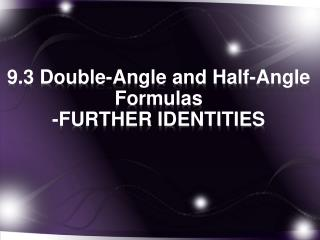DownloadDownload Presentation9.3 Double-Angle and Half-Angle Formulas -FURTHER IDENTITIES

# 9.3 Double-Angle and Half-Angle Formulas -FURTHER IDENTITIES

Télécharger la présentation## 9.3 Double-Angle and Half-Angle Formulas -FURTHER IDENTITIES

- - - - - - - - - - - - - - - - - - - - - - - - - - - E N D - - - - - - - - - - - - - - - - - - - - - - - - - - -
##### Presentation Transcript

1. 9.3 Double-Angle and Half-Angle Formulas -FURTHER IDENTITIES

2. Double Angle Formulas • Suppose we use the sum formula for sine, but the two values that we add are the same, that is α=β

3. Double angle formula for cosine

4. Alternate forms of the cosine double angle formula Because we know that sin2x+cos2x=1, we can alter that equation and solve for sin2x or cos2x and make substitutions into the double angle formula.

5. Now do the same thing but lets substitute for sin2x instead.

6. Double-angle Formula for Tangent Hwk, pg. 383 1-16, 19, 20

7. 9.3 Finding Function Values of 2 Example Given and sin  < 0, find sin 2, cos 2, and tan 2. Solution To find sin 2, we must find sin . Choose the negative square root since sin  < 0.

8. 9.3 Finding Function Values of 2

9. 9.3 Simplifying Expressions Using Double-Number Identities Example Simplify each expression. • cos² 7x – sin² 7x (b) sin 15° cos 15° Solution • cos 2A = cos² A – sin² A. Substituting 7x in for A gives cos² 7x – sin² 7x = cos 2(7x) = cos 14x. • Apply sin 2A = 2 sin A cos A directly.

10. 9.3 Half-Number Identities • Half-number or half-angle identities for sine and cosine are used in calculus when eliminating the xy-term from an equation of the form Ax² + Bxy + Cy² + Dx + Ey + F = 0, so the type of conic it represents can be determined. • From the alternative forms of the identity for cos 2A, we can derive three additional identities, e.g. Choose the sign ± depending on the quadrant of the angle A/2.

11. 9.3 Half-Number Identities Half-Number Identities

12. 9.3 Using a Half-Number Identity to Find an Exact Value Example Find the exact value of Solution

13. 9.3 Finding Function Values of x/2 Example Given Solution The half-angle terminates in quadrant II since

14. 9.3 Simplifying Expressions Using Half-Number Identities Example Simplify the expression Solution This matches the part of the identity for cos A/2. Replace A with 12x to get

15. HWK pg. 383 1-12 • HWK pg. 384 19-22, 31-36